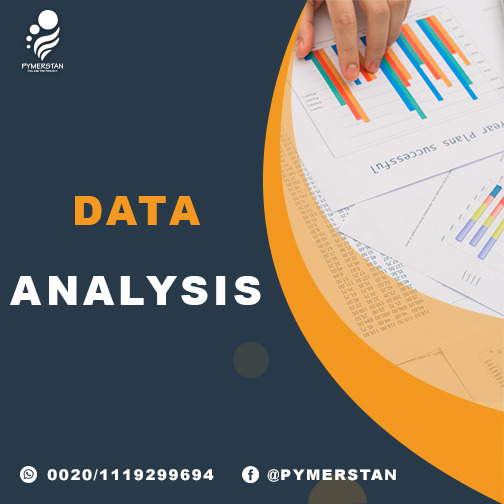# Data Analysis Course164 \$

30 lectures

2 months

## Objective

#### 1.

Basic statistics.

Types of data.

Scale of data.

Data conversion.

#### 5.

Descriptive statistics.

#### 6.

The Gaussian distribution.

#### 7.

The empirical rule.

#### 8.

Normality & non-normality.

#### 9.

Data visualization & graphing.

#### 10.

Inferential statistics.

Data collection.

Sampling.

#### 13.

The central limit theorem.

#### 14.

Test of hypothesis for all types of data.

Scatter plot.

Correlation.

#### 17.

Simple regression.

#### 18.

Polynomial regression.

#### 19.

Multiple linear regression.

#### 20.

Confidence intervals & prediction intervals.

#### 21.

Statistical process control (Control charts).

#### 22.

Examples & exercises for all the topics on Minitab statistical software.

# Interest with this course.#### Pymerstan

Medical Advertising ,Marketing , Training , Consultation and Other coming